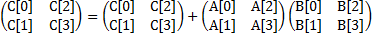## Lab 9

Computer Architecture I ShanghaiTech University
Lab 8 Lab 9 Lab 10

## Setup

Note that we are using SSE and SSE2 in this lab, since they are enabled by default in GCC on x86-64 platforms. If your CPU does not support SSE and SSE2 (which is very not likely), try to switch to one that supports.

## Exercises

### Exercise 1: Familiarize Yourself

Given the large number of available SIMD intrinsics we want you to learn how to find the ones that you'll need in your application.

Open Intel Intrinsics Guide. Do your best to interpret the new syntax and terminology. Find the 128-bit intrinsics for the following SIMD operations (one for each):

• Four floating point divisions in single precision (i.e. float)
• Sixteen max operations over signed 8-bit integers (i.e. char)
• Arithmetic shift right of eight signed 16-bit integers (i.e. short)

#### Checkoff

• Record these intrinsics in a text file to show your TA.

### Exercise 2: Reading SIMD Code

In this exercise you will consider the vectorization of 2-by-2 matrix multiplication in double precision:This accounts to the following arithmetic operations:

```    C += A*B + A*B;
C += A*B + A*B;
C += A*B + A*B;
C += A*B + A*B;
```

You are given the code sseTest.c that implements these operations in a SIMD manner.
The following intrinsics are used:

 __m128d _mm_loadu_pd( double *p ) returns vector (p, p) __m128d _mm_load1_pd( double *p ) returns vector (p, p) __m128d _mm_add_pd( __m128d a, __m128d b ) returns vector (a0+b0, a1+b1) __m128d _mm_mul_pd( __m128d a, __m128d b ) returns vector (a0b0, a1b1) void _mm_storeu_pd( double *p, __m128d a ) stores p=a0, p=a1

Compile sseTest.c into x86 assembly by running:

```make sseTest.s
```

Find the for-loop in sseTest.s and identify what each intrinsic is compiled into. Does the loop actually exist? Comment the loop so that your TA can see that you understand the code.

#### Checkoff

• Show your commented code to your TA and explain the for-loop.

### Exercise 3: Writing SIMD Code

For Exercise 3, you will vectorize/SIMDize the following code to achieve approximately 4x speedup over the naive implementation shown here:

```   static int sum_naive(int n, int *a)
{
int sum = 0;
for (int i = 0; i < n; i++)
{
sum += a[i];
}
return sum;
}
```

You might find the following intrinsics useful:

 __m128i _mm_setzero_si128( ) returns 128-bit zero vector __m128i _mm_loadu_si128( __m128i *p ) returns 128-bit vector stored at pointer p __m128i _mm_add_epi32( __m128i a, __m128i b ) returns vector (a0+b0, a1+b1, a2+b2, a3+b3) void _mm_storeu_si128( __m128i *p, __m128i a ) stores 128-bit vector a at pointer p

To compile your code, run the following command:

`make sum`

### Exercise 4: Loop Unrolling

Happily, you can obtain even more performance improvement! Carefully unroll the SIMD vector sum code that you created in the previous exercise. This should get you about a factor of 2 further increase in performance. As an example of loop unrolling, consider the supplied function sum_unrolled():

```   static int sum_unrolled(int n, int *a)
{
int sum = 0;

// unrolled loop
for (int i = 0; i < n / 4 * 4; i += 4)
{
sum += a[i+0];
sum += a[i+1];
sum += a[i+2];
sum += a[i+3];
}

// tail case
for (int i = n / 4 * 4; i < n; i++)
{
sum += a[i];
}

return sum;
}
```

Also, feel free to check out Wikipedia's article on loop unrolling for more information.

Within sum.c, copy your sum_vectorized() code into sum_vectorized_unrolled() and unroll it four times.

To compile your code, run the following command:

```make sum
```

#### Checkoff:

• Show your TA the unrolled implementation and performance improvement.

### Exercise 5: Switch on Compiler Optimization

Modify the Makefile to activiate the compiler optimization (e.g. -O2)

To compile your code, run the following command:

```make sum
```

#### Checkoff:

• Show your TA the performance of the compiler optimized code. Explain the results.